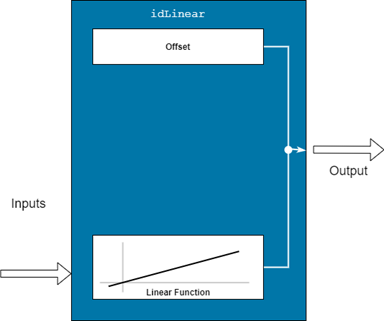# idLinear

Linear mapping object for nonlinear ARX models

## Description

An `idLinear` object implements an affine function, and is a mapping function for estimating nonlinear ARX models. The mapping function uses a combination of linear weights and an offset. Unlike the other mapping objects for the nonlinear models, the `idLinear` object contains no accommodation for a nonlinear component.Mathematically, `idLinear` is a linear function $y=F\left(x\right)$ that maps m inputs X(t) = [x(t1),x2(t),…,xm(t)]T to a scalar output y(t). . F is a (affine) function of x:

`$y\left(t\right)={y}_{0}+{\left(Χ\left(t\right)-\overline{X}\right)}^{T}PL$`

Here:

• X(t) is an m-by-1 vector of inputs, or regressors, with mean $\overline{Χ}$.

• y0 is the output offset, a scalar.

• P is an m-by-p projection matrix, where m is the number of regressors and is p is the number of linear weights. m must be greater than or equal to p.

• L is a p-by-1 vector of weights.

Set `idLinear` as the value of the `OutputFcn` property of an `idnlarx` model. For example, specify `idLinear` when you estimate an `idnlarx` model with the following command.

`sys = nlarx(data,regressors,idLinear)`
When `nlarx` estimates the model, it also estimates the parameters of the `idLinear` function.

Use the `idLinear` mapping object when you want to create nonlinear ARX models that operate linearly on the regressors. The regressors themselves can be nonlinear functions of the inputs and outputs. The `polynomialRegressor` and `customRegressor` commands allow you to create such regressors. When the `idnlarx` model has no custom regressors and the output function is set to `idLinear`, the model is similar to a linear ARX model. However, for the nonlinear ARX model, the offset is an estimable parameter.

You can configure the `idLinear` object to disable components and fix parameters. Use `evaluate` to compute the output of the function for a given vector of inputs.

## Creation

### Syntax

``Lin = idLinear``

### Description

example

````Lin = idLinear` creates an `idLinear` object `Lin` with unknown parameters. ```

## Properties

expand all

Input signal information for signals used for estimation, specified as vectors of m property-specific values, where m is the number of input signals. The `Input` properties for each input signal are as follows:

• `Name` — Names of the input signals, specified as a 1-by-m string or character array, where m is the number of inputs

• `Mean` — Mean of the input signals, specified as a numeric scalar

• `Range` — Ranges of the input signals, specified as a 2-by-m numeric array that contains the minimum and maximum values

Output signal information, specified as property-specific values. The `Output` properties are as follows:

• `Name` — Name of the output signal, specified as a string or a character array

• `Mean` — Mean of the output signal, specified as a numeric scalar

• `Range` — Range of the output signal, specified as 2-by-1 numeric array that contains the minimum and maximum values.

Parameters of the linear function, specified as follows:

• `Value` — Value of L', specified as a 1-by-m vector.

• `Free` — Option to update entries of `Value` during estimation. specified as a logical scalar. The software honors the `Free` specification only if the starting value of `Value` is finite. The default value is `true`.

• `Minimum` — Minimum bound on `Value`, specified as a 1-by-p vector. If `Minimum` is specified with a finite value and the starting value of `Value` is finite, then the software enforces that minimum bound during model estimation.

• `Maximum` — Maximum bound on `Value`, specified as a 1-by-p vector. If `Maximum` is specified with a finite value and the starting value of `Value` is finite, then the software enforces that maximum bound during model estimation.

• `SelectedInputIndex` — Indices of `idLinear` inputs (see `Input.Name`) that are used as inputs to the linear function, specified as an 1-by-nr integer vector, where nr is the number of inputs. The `RegressorUsage` property of the `idnlarx` model determines these indices.

Parameters of the offset term, specified as follows:

• `Value` — Offset value, specified as a scalar.

• `Free` — Option to update `Value` during estimation, specified as a scalar logical. The software honors the `Free` specification of `false` only if the value of `Value` is finite. The default value is `true`.

• `Minimum` — Minimum bound on `Value`, specified as a numeric scalar or `–Inf`. If `Minimum` is specified with a finite value and the value of `Value` is finite, then the software enforces that minimum bound during model estimation. The default value is `-Inf`.

• `Maximum` — Maximum bound on `Value`, specified as a numeric scalar or `Inf`. If `Maximum` is specified with a finite value and the starting value of `Value` is finite, then the software enforces that maximum bound during model estimation. The default value is `Inf`.

## Examples

collapse all

`load iddata7 z7`

Create an `idLinear` mapping object `L`.

`L = idLinear;`

Create model regressors that include nonlinear polynomial regressors.

```Reg1 = linearRegressor({'y1','u1'},{1:4, 0:4}); Reg2 = polynomialRegressor({'y1','u1'},{1:2, 0:2},2,false,true,true); Reg3 = polynomialRegressor({'y1','u1'},{2, 1:3},3,false,true);```

Estimate the nonlinear ARX model.

`sys = nlarx(z7,[Reg1;Reg2;Reg3],L)`
```sys = Nonlinear ARX model with 1 output and 2 inputs Inputs: u1, u2 Outputs: y1 Regressors: 1. Linear regressors in variables y1, u1 2. Order 2 regressors in variables y1, u1 3. Order 3 regressors in variables y1, u1 List of all regressors Output function: None Sample time: 1 seconds Status: Estimated using NLARX on time domain data "z7". Fit to estimation data: 43.22% (prediction focus) FPE: 5.66, MSE: 4.963 ```

## Compatibility Considerations

expand all

Not recommended starting in R2021b

Not recommended starting in R2021a

Introduced in R2007a

## SupportGet trial now